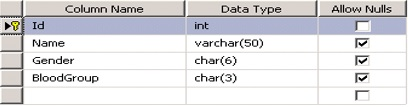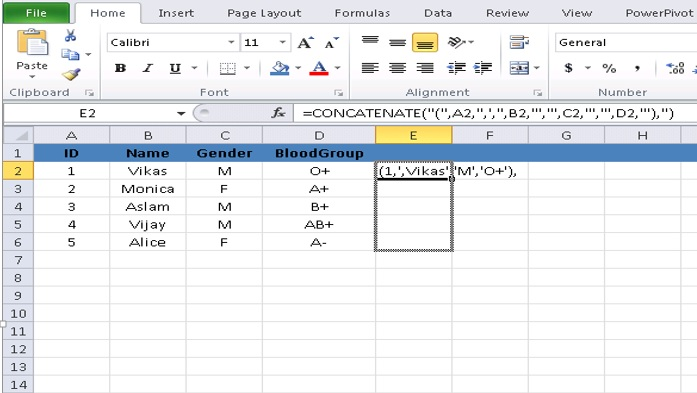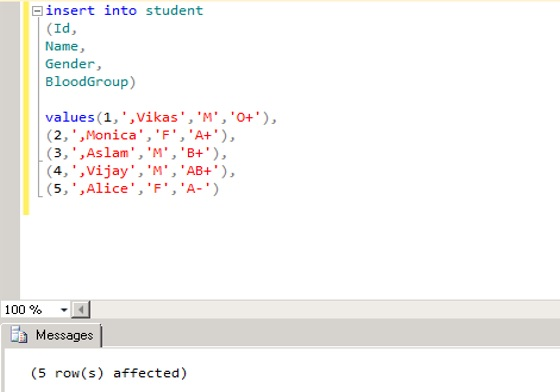# How to import data from excel to SQL Server Table by generating SQL Script in Excel

Although there are multiple ways to import data from excel to SQL Server tables. But I am explaining you a method by which you can generate the SQL script from excel file and that script you can use at many different servers like Test, Development and Production Server etc.

Let us consider a Student Table as shown belowLet us consider the excel sheet as shown below. I want to import data from excel sheet into SQL Server Table.I am writing a CONCATENATE function in the cell E2 of excel as shown below. CONCATENATE function will concatenate all the comma separated data into a single string. String values are represented in double quotes. A2, B2, C2 AND D2 are the cell values for the second row in excel.Hit enter to generate the script as shown below.Drag from cell E2 till E6 to generate the SQL script as shown below.SQL script has been generated in excel as shown below.Another advantage is that you can save this formula that you have created in excel for future use.

Now copy the excel script and paste it in SSMS. Use the following script with the insert command to insert the data into the tables.